## Time Average

There is a better way to compute average of a sequence of large measurement data. You don�t need to store all the measurement data. All you need is to compute the current average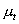is the current measurement data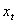and the previous average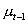. The formula is as follow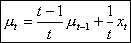(3)

Since the subscript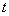start at 1, thenis undefined. For convenient, we can put zero (or any number) to give the correct answer.

Using the previous measurement data of 4, 6, 12, 9 we can get exactly the same result of average as the usual computation method, but in more efficient way. Table below show the computation using the recursive average.

 Time () Measurement () Average () 1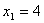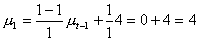2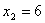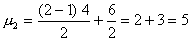3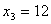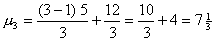4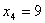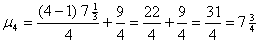The interactive program below you may type your input (only number is accepted) one at a time, then press "Input" button. To compute the time average based on your current available data. Click "Reset" button to restart. Alternatively, click "Random Input" button repeatedly to create simulated data. You need to input at least two data points to make the chart.

Current input data

Current Average:
0

Previous Average:
0

Number of data:
0